# Gate in Computer Science | A Basic Logic Gate Reference for those new to CS

Logic gates are the essential building blocks for digital circuits.

Each Terminal of a logic gate will always be in one of two binary states, either a (0) or (1).

These binary states are simply the presence (1), or absence (0) of an electrical voltage. You may also see the presence of voltage (1) referred to as ON or High. Whereas the absence of voltage (0) may be referred to as OFF or Low.

Here are the 7 basic logic gates alongside their notations and explanations.

## The 7 Basic Logic Gates in Computer Science

The seven basic logic gates are AND, OR, NOR, XOR, NOT, NAND, and XNOR.

In the following Gate cheat sheet, the terminals are noted as A or B or a combination of the two.Quick tip:

• ____ above notation = Not
• encircling the + = eXclusive

Every possible binary input combination of these logic gates can be represented in what's called a Truth Table.

Here's the Truth Table for all of the basic logic gates you'll find in Computer Science.

## Basic logic gates represented using the Truth Table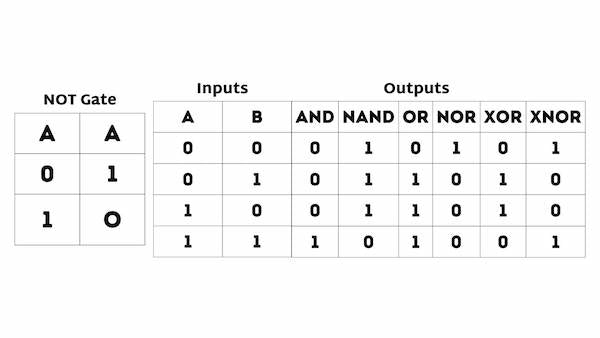Now let's get into each gate with a little more depth.

### NOT Gate

The NOT Gate is also known as an inverter because it simply accepts a single input and outputs the opposite value. If the input is low (0), the output is high (1), and vice versa.The NOT gate plays a crucial role in digital logic design as it can be used to negate or flip certain signals, leading to more complex operations and circuits.

NOT gate tips

• If the input variable is A, the inverted output is known as NOT A.  This is also shown as A', or A with a bar over the top, as shown at the outputs.### AND Gate

The AND gate has two inputs, but only outputs a high signal (1) if both inputs are high (again, both 1s). Otherwise, it outputs a low signal (0).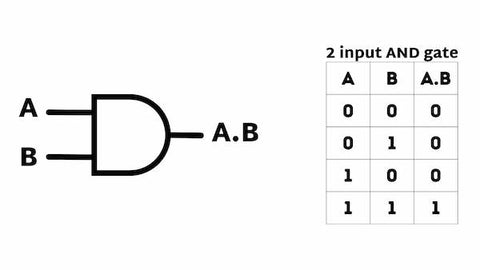The And Gate is often used in combination with other logic gates to create more complex circuits and perform more intricate operations.

AND gate tips
- A dot (.) is used to show the AND operation i.e. A.B.  Bear in mind that this dot is sometimes omitted i.e. AB

### NAND Gate

The NAND gate stands for Not AND.

It's like an AND gate with an inverted output, meaning it outputs a low signal (0) only when both inputs are high. Otherwise, it outputs a high signal (1).### OR Gate

The OR Gate also has two inputs, but outputs a high signal (1) if either of its inputs are high. If both inputs are low, it outputs a low signal (0).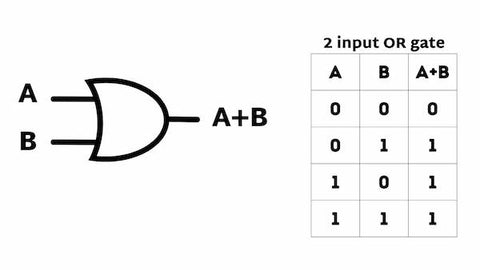OR gate tips

- A plus (+) is used to show the OR operation.

### NOR Gate

The sixth type is the NOR gate, which stands for NOT OR.

It's like an OR gate with an inverted output, meaning it outputs a low signal (0) only if either input is high. If both inputs are low, it outputs a high signal (1).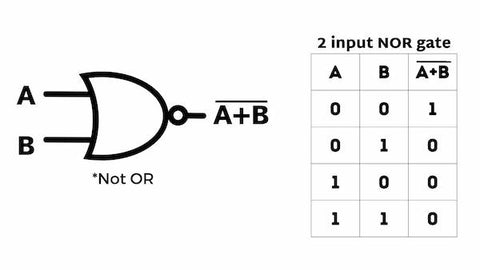### XOR Gate

The XOR gate stands for eXclusive OR.

This gate has two inputs and outputs a high signal (1) if only one of the inputs is high. If both inputs are high or low, it outputs a low signal (0).Here's an example of the XOR Gate, which we used in Computer Engineering for Babies.### XNOR Gate

Finally, we have the XNOR gate, which stands for eXclusive Not OR.

This gate is like a combination of the XOR and the NOR gate. It outputs a high signal (1) only when both inputs are the same (either both high or both low). Otherwise, it outputs a low signal (0).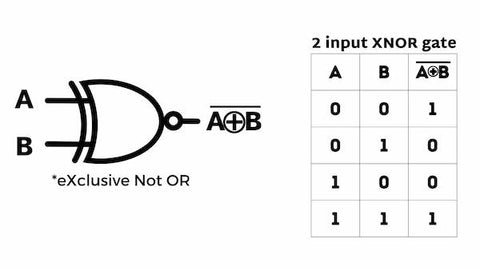## Gate in Computer Science refresher

If you’re looking to get into the computer science field, you’ll need to know these basic logic gates like the back of your hand.

To help you stay fresh, we made a book that explores 4 of the basic logic gates; AND, OR, XOR, NOT as well as a Wire and a Latch, it's called Computer Engineering for Babies.